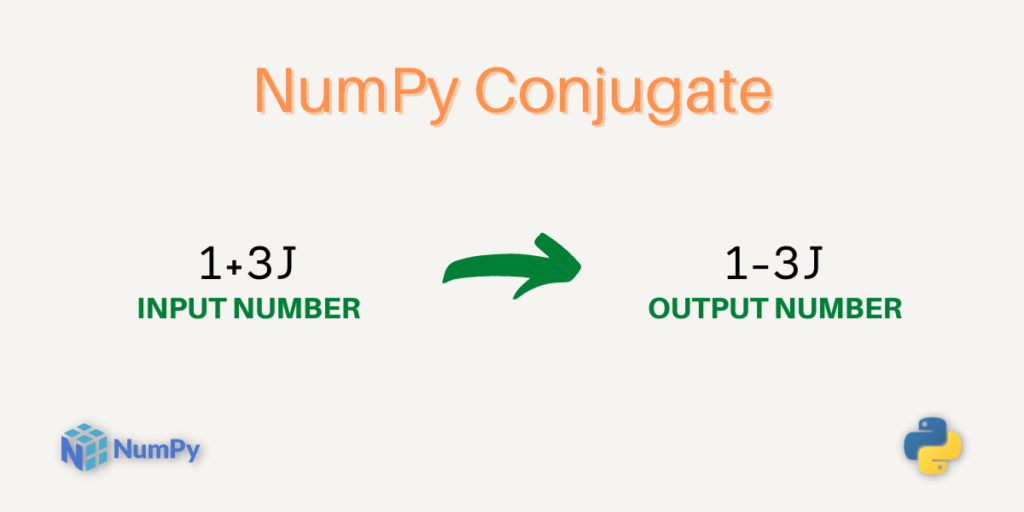# NumPy conj – Return the Complex Conjugate of an Input NumberHey everyone, welcome to another NumPy mathematical functions tutorial. In this tutorial, we will understand the `NumPy conj` function in detail.

The conjugate of a complex number is obtained by simply changing the sign of the imaginary part.

For example, the conjugate of a complex number 10-8j is 10+8j. We can obtain the conjugate of a complex number using the `numpy.conj()` function.

So, let’s get started.

Also read: NumPy fmax – Element-wise maximum of array elements

NumPy conj is a mathematical function of the numpy library that calculates the complex conjugate of the input complex numbers.

### Syntax

By definition, it sounds simple right? Now, let us look at the syntax of the function.

```numpy.conj(input)
```

Here, the input can be a single complex number as well as a NumPy array of complex numbers.

## Working with NumPy conj

Now, let’s do some programming in python.

### NumPy conj of a single complex number

```import numpy as np

# Complex Conjugate of a Complex number with real and imaginary parts
print("The complex conjugate of 1+6j is:",np.conj(1+6j))
print("The complex conjugate of 1-6j is:",np.conj(1-6j))

# Complex Conjugate of a Complex number with only imaginary part
print("The complex conjugate of 0+6j is:",np.conj(0+6j))
print("The complex conjugate of 0-6j is:",np.conj(0-6j))

# Complex Conjugate of a Complex number with only real part
print("The complex conjugate of 1 is:",np.conj(1))
print("The complex conjugate of -1 is:",np.conj(-1))
```

### Output

```The complex conjugate of 1+6j is: (1-6j)
The complex conjugate of 1-6j is: (1+6j)
The complex conjugate of 0+6j is: -6j
The complex conjugate of 0-6j is: 6j
The complex conjugate of 1 is: 1
The complex conjugate of -1 is: -1
```

In the above snippet, the NumPy library is imported using the `import` statement and the function `np.conj()` is used to calculate the complex conjugate of the input complex number.

Let’s understand how the values are calculated.

For complex number `1+6j`, the conjugate is obtained by changing the sign of the imaginary part and hence the output is `1-6j`.

For complex number `1`, the conjugate will be the same as the input complex number. This is because the number 1 can be written as `1+0j`, where the imaginary part is 0, hence the output is the same as the input complex number.

Now, let us pass a NumPy array of complex numbers and calculate the complex conjugate.

### NumPy conj of a NumPy array of complex numbers

```import numpy as np

a = np.array((1+3j , 0+6j , 5-4j))

b = np.conj(a)

print("Input Array:\n",a)
print("Output Array:\n",b)
```

Output

```Input Array:
[1.+3.j 0.+6.j 5.-4.j]
Output Array:
[1.-3.j 0.-6.j 5.+4.j]
```

In the above snippet, a NumPy array of complex numbers is created using the `np.array()` which is stored in the variable `a`. The variable `b` stores the conjugate values of the input array which is also a NumPy array.

The `np.conj()` calculates the conjugate of each element of the input array.

In the next lines, we have used print statements to print the Input Array and Output Array.

### NumPy conj of a NumPy array using the NumPy eye function

In this code snippet, we will create a NumPy array using the `numpy.eye()`.

```import numpy as np

a = np.eye(2) + 1j * np.eye(2)

b = np.conj(a)

print("Input Array:\n",a)
print("Conjugated Values:\n",b)
```

Output

```Input Array:
[[1.+1.j 0.+0.j]
[0.+0.j 1.+1.j]]
Conjugated Values:
[[1.-1.j 0.-0.j]
[0.-0.j 1.-1.j]]
```

Let’s try to understand the above code snippet.

• In the first line, we import the NumPy library using the `import` statement.
• The function `np.eye(2)` creates a 2×2 array with the diagonal elements as 1 and other elements as 0.
• Similarly, the expression `1j * np.eye(2)` creates a 2×2 array with the diagonal elements as 1j and other elements as 0.
• Then, the expression `np.eye(2)` `+` `1j * np.eye(2)` adds the corresponding elements of the two arrays, which is stored in the variable a.
• In the next line, we used the `np.conj()` function to calculate the conjugated values.

That was all about using the NumPy conj function.

## Summary

In this tutorial, you learned about the NumPy conj function along with practicing different types of examples. There is one more function `numpy.conjugate()` that works exactly the same way as the `numpy.conj()` function. Happy learning and keep coding.

## Reference

NumPy Documentation – NumPy conj